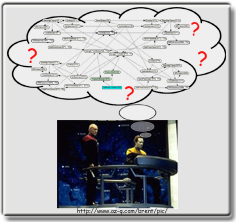Multi-Entity Bayesian Networks

MEBN: A Bayesian First-Order LogicWhat is it?

A logic system that integrates First Order Logic (FOL) with Bayesian probability theory. MEBN extends ordinary Bayesian networks to allow representation of graphical models with repeated sub-structures.  Knowledge is encoded as a collection of Bayesian network fragments (MFrags) that can be instantiated and combined to form highly complex situation-specific Bayesian networks. A MEBN theory (MTheory) implicitly represents a joint probability distribution over possibly unbounded numbers of hypotheses, and uses Bayesian learning to refine a knowledge base as observations accrue.  MEBN provides a logical foundation for the emerging collection of highly expressive probability-based languages.

MEBN in a Nutshell

Multi-Entity Bayesian Networks (Laskey, 2008) integrate first order logic with Bayesian probability. MEBN logic expresses probabilistic knowledge as a collection of MEBN fragments (MFrags) organized into MEBN Theories (MTheories). An MFrag represents a conditional probability distribution of the instances of its resident random variables given the values of instances of their parents in the Fragment graphs and given the context constraints.

A collection of MFrags represents a joint probability distribution over an unbounded, possibly infinite number of instances of its random variables. The joint distribution is specified by means of the local distributions together with the conditional independence relationships implied by the fragment graphs. Context terms are used to specify constraints under which the local distributions apply.

A collection of MFrags that satisfies consistency constraints ensuring the existence of a unique joint probability distribution over its random variables is called an MTheory. MTheories can express probability distributions over truth values of arbitrary First Order Logic sequences and can be used to express domain-specific ontologies that capture statistical regularities in a particular domain of application.

In addition, MTheories can represent particular facts relevant to a given reasoning problem. Conditioning a prior distribution represented by an MTheory on its findings is the basis of probabilistic inference with MEBN logic.

Support for Decisions

Support for decision constructs in MEBN is provided via Multi-Entity Decision Graphs (MEDG), which are related to MEBN the same way influence diagrams are related to Bayesian Networks. An MEDG can be applied in any application that requires optimizing a set of alternatives (i.e. an MEDG policy) over the given constraints of a specific situation. MEBN logic also provides means of learning the structure of a MEBN Theory on the basis of data (i.e. Bayesian learning), while parameter learning can be expressed as inference in MEBN theories that contain parameter random variables.

References

Laskey, K. B. (2008) MEBN: A Language for First-Order Bayesian Knowledge Bases. Artificial Intelligence, 172(2-3).